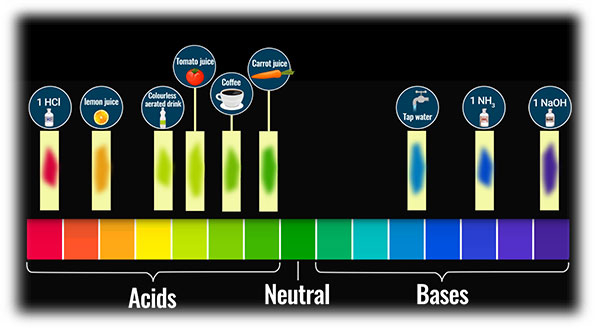# On adding a few drops of universal indicator solution to three unknown colourless solutions P, Q, and R taken separately in three test-tubes shown in the diagrams, a student observed the changes in colour as green in solution P, red solution Q and violet in solution R.The decreasing order of the pH of the three solution is :(1) P > Q > R(2) R > P > Q(3) Q > P > R (4) R > Q > P"

Correct answer:  (2) R > P > Q

Explanation:From the above image of universal indicator, as P shows green colour, so it is neutral, Q shows red colour, so it is an acid and R shows violet colour, so it is a base.

So, from the above discussion, we can arrange the given molecules in decreasing order as R > P > Q.